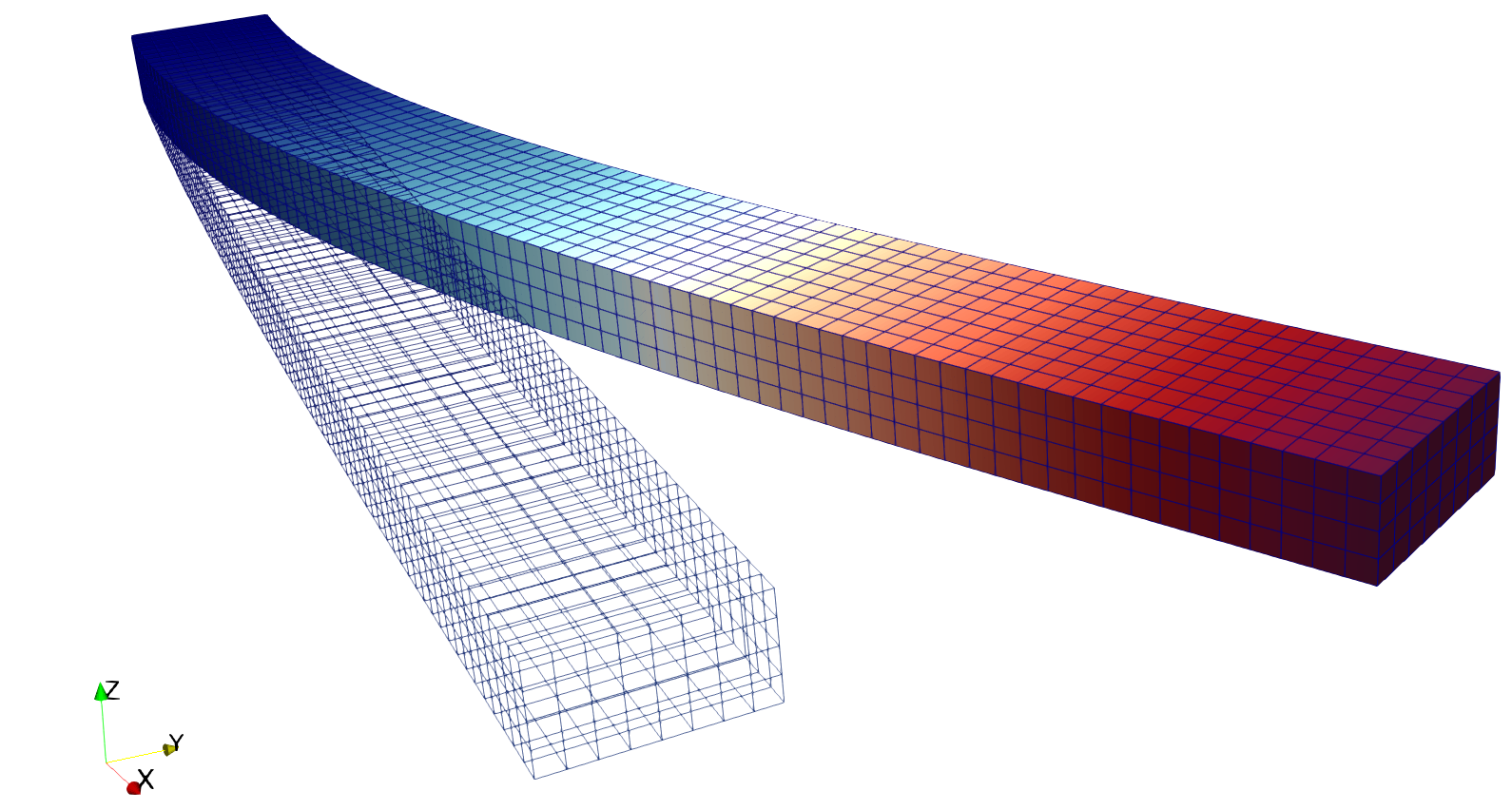# Tutorial 4: Boundary Conditions and Time-Dependent Functions¶

Context

In this tutorial, we use a small strain linear elastic based solid mechanics solver (see Solid Mechanics Solver) from GEOS to solve for the bending problem of a three-dimensional cantilever beam. The beam is fixed at one end, and subjects to a traction force pointing to the y-positive direction on the other end. The beam is deformed in the x-y plane.

Objectives

At the end of this tutorial, you will know:

• how to use the solid mechanics solver to solve a quasistatic problem,

• how to set up displacement boundary condition at element nodes,

• how to set up traction boundary condition on element surfaces,

Input file

This tutorial uses no external input files and everything required is contained within a single GEOS input file. The xml input file for this test case is located at:

```inputFiles/solidMechanics/beamBending_base.xml
inputFiles/solidMechanics/beamBending_benchmark.xml
```

## Discretized computational domain¶

The following mesh is used in this tutorial:This mesh contains 80 x 8 x 4 eight-node brick elements in the x, y and z directions, respectively. Here, the `InternalMesh` is used to generate a structured three-dimensional mesh with `C3D8` as the `elementTypes`. This mesh is defined as a cell block with the name `cb1`.

```  <Mesh>
<InternalMesh
name="mesh1"
elementTypes="{ C3D8 }"
xCoords="{ 0, 80 }"
yCoords="{ 0, 8 }"
zCoords="{ 0, 4 }"
nx="{ 160 }"
ny="{ 16 }"
nz="{ 8 }"
cellBlockNames="{ cb1 }"/>
</Mesh>
```

## Gravity¶

The gravity is turned off explicitly at the beginning of the input file:

```  <Solvers
gravityVector="{ 0.0, 0.0, 0.0 }">
```

## Solid mechanics solver¶

The solid mechanics solver is based on the small strain Lagrangian finite element formulation. The problem is run as `QuasiStatic` without considering the beam inertial. The computational domain is discretized by `FE1`, which is defined in the `NumericalMethods` block. The material is designated as `shale`, whose properties are defined in the `Constitutive` block.

```    <SolidMechanicsLagrangianSSLE
name="lagsolve"
timeIntegrationOption="QuasiStatic"
discretization="FE1"
targetRegions="{ Region2 }"
logLevel="1">
```

## Finite element discretization¶

The computational domain is discretized by `C3D8` elements with the first order interpolation functions at each direction in the parent domain. The 2 x 2 x 2 Gauss quadrature rule is adopted to be compatible with the first order interpolation functions.

## Constitutive model¶

Recall that in the `SolidMechanicsLagrangianSSLE` block, `shale` is designated as the material in the computational domain. Here, the material is defined as linear isotropic.

```    <ElasticIsotropic
name="shale"
defaultDensity="2700"
defaultBulkModulus="5.5556e9"
defaultShearModulus="4.16667e9"/>
```

## Boundary conditions¶

As aforementioned, the beam is fixed on one end, and subjects to surface traction on the other end. These boundary conditions are set up through the `FieldSpecifications` block. Here, `nodeManager` and `faceManager` in the `objectPath` indicate that the boundary conditions are applied to the element nodes and faces, respectively. Component `0`, `1`, and `2` refer to the x, y, and z direction, respectively. And the non-zero values given by `Scale` indicate the magnitude of the loading. Some shorthands, such as `xneg` and `xpos`, are used as the locations where the boundary conditions are applied in the computational domain. For instance, `xneg` means the portion of the computational domain located at the left-most in the x-axis, while `xpos` refers to the portion located at the right-most area in the x-axis. Similar shorthands include `ypos`, `yneg`, `zpos`, and `zneg`. Particularly, the time-dependent loading applied at the beam tip is defined through a function with the name `timeFunction`.

```  <FieldSpecifications>
<FieldSpecification
name="xnegconstraint"
objectPath="nodeManager"
fieldName="totalDisplacement"
component="0"
scale="0.0"
setNames="{ xneg }"/>

<FieldSpecification
name="yconstraint"
objectPath="nodeManager"
fieldName="totalDisplacement"
component="1"
scale="0.0"
setNames="{ xneg }"/>

<FieldSpecification
name="zconstraint"
objectPath="nodeManager"
fieldName="totalDisplacement"
component="2"
scale="0.0"
setNames="{ zneg, zpos }"/>

<Traction
name="xposconstraint"
objectPath="faceManager"
scale="1.0e6"
direction="{ 0, 1, 0 }"
functionName="timeFunction"
setNames="{ xpos }"/>
</FieldSpecifications>
```

## Table function¶

A table function is used to define the time-dependent loading at the beam tip. The `coordinates` and `values` form a time-magnitude pair for the loading time history. In this case, the loading magnitude increases linearly as the time evolves.

```  <Functions>
<TableFunction
name="timeFunction"
inputVarNames="{ time }"
coordinates="{ 0.0, 10.0 }"
values="{ 0.0, 10.0 }"/>
</Functions>
```

## Execution¶

Finally, the execution of the simulation is set up in the `Events` block, where `target` points to the solid mechanics solver defined in the `Solvers` block, and the time increment `forceDt` is set as 1.0s.

```    <PeriodicEvent
name="solverApplications"
forceDt="1.0"
target="/Solvers/lagsolve"/>
```

## Result¶

The deformed beam is shown as following (notice that the displacement is visually magnified):## To go further¶

Feedback on this tutorial

This concludes the solid mechanics for small-strain linear elasticity tutorial. For any feedback on this tutorial, please submit a GitHub issue on the project’s GitHub page.

For more details